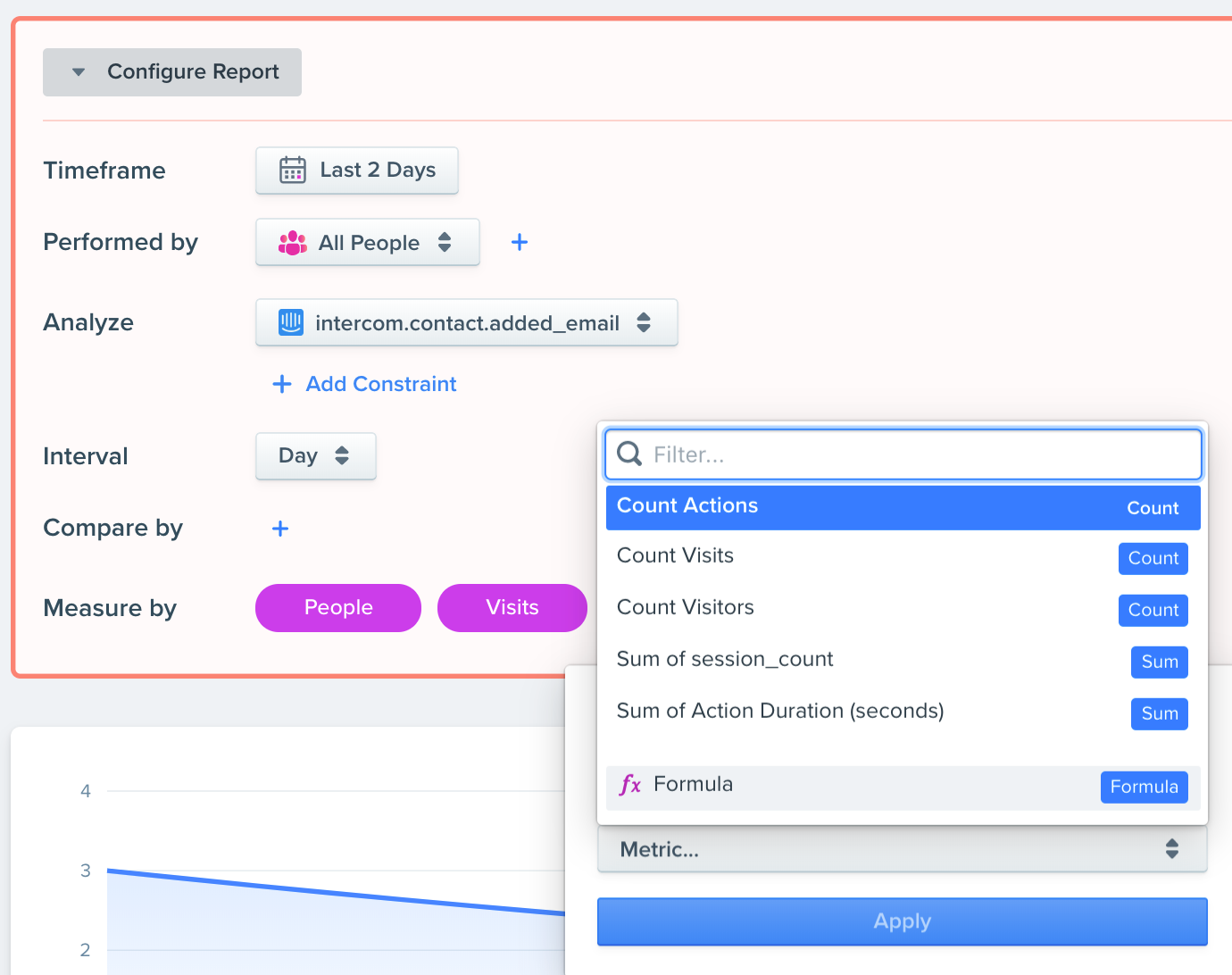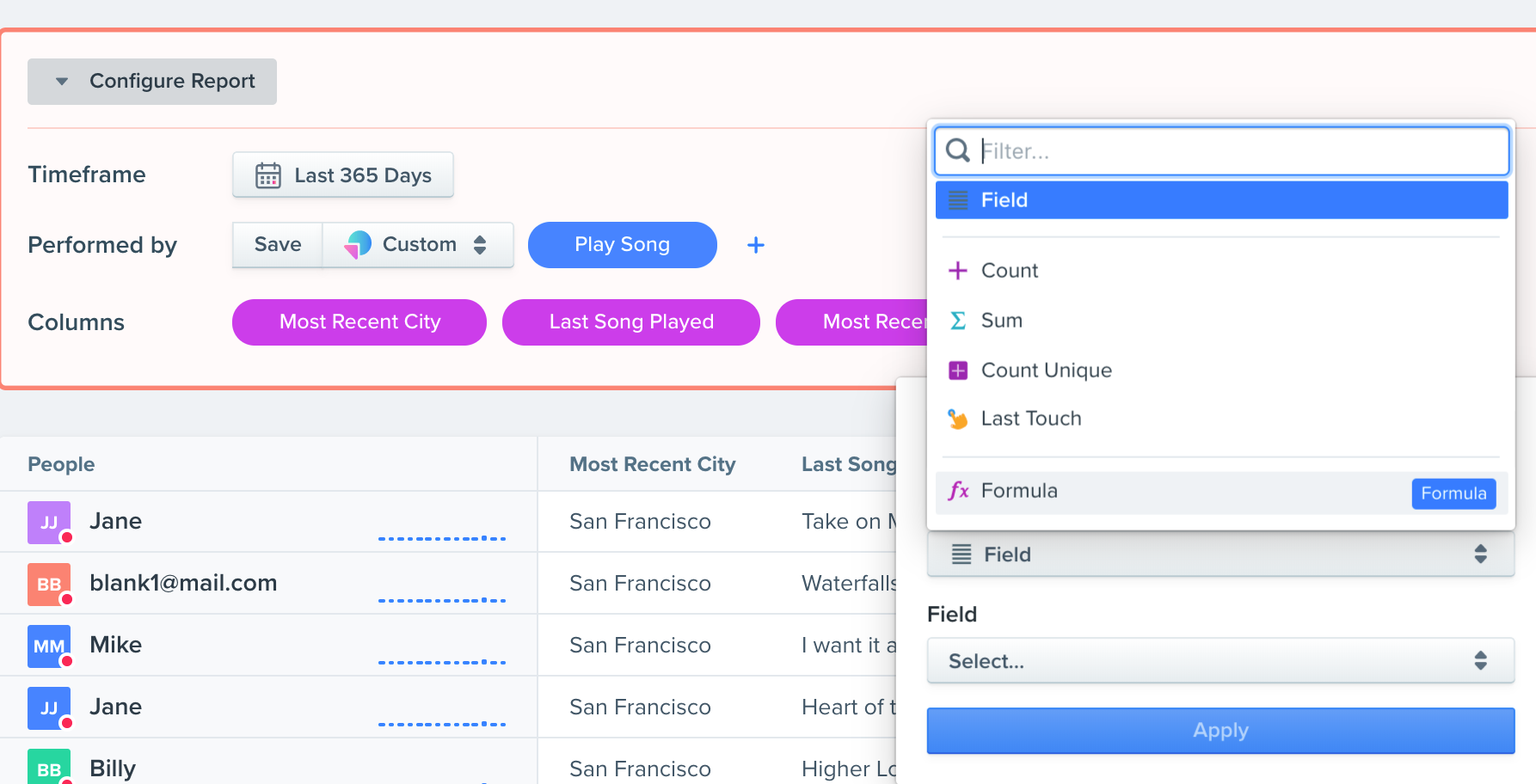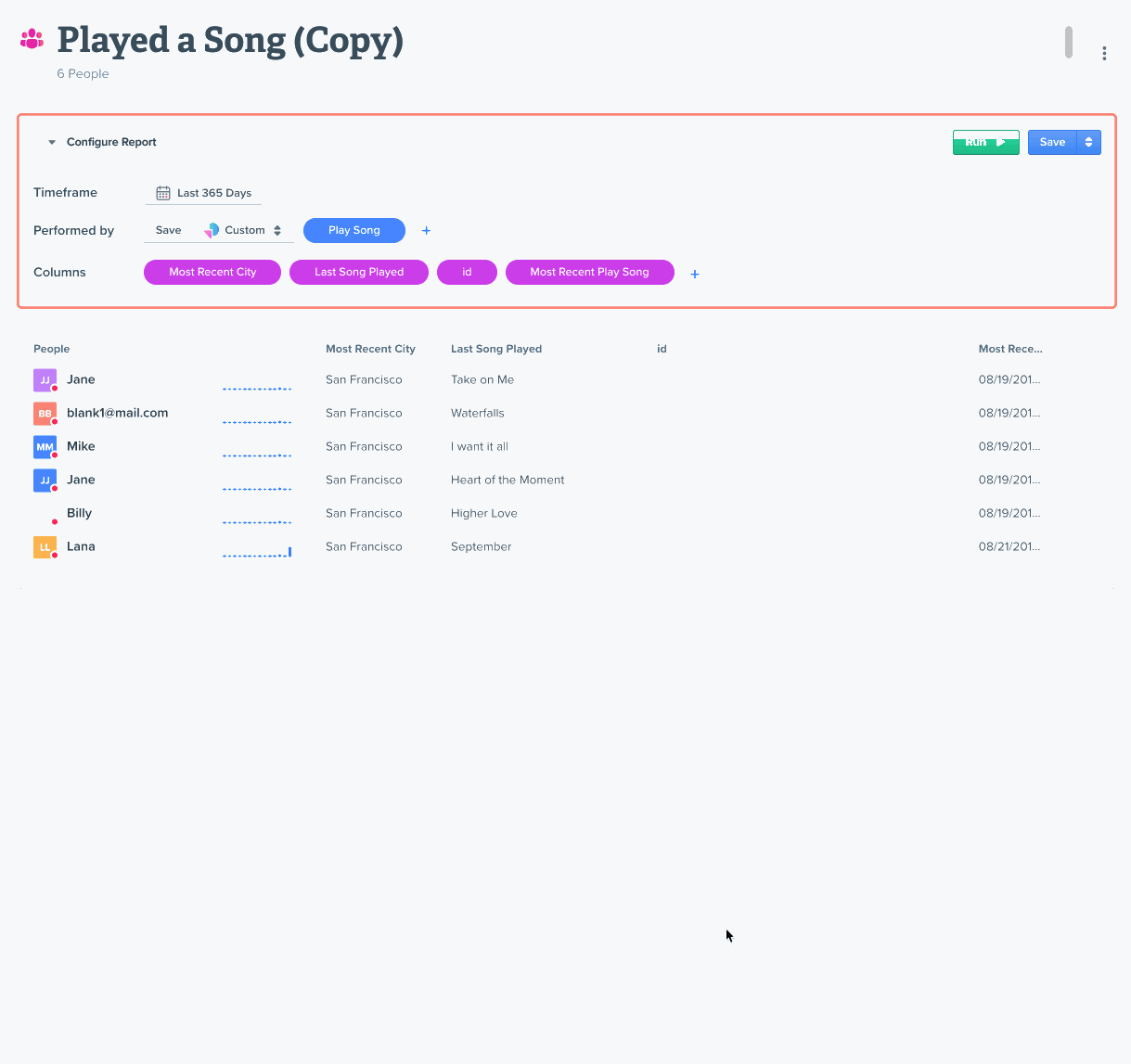# Overview

Woopra formulas allow you to do row-based calculations in Trend and People reports. You can also use formulas to create Custom Action Schema Properties and Custom Visitor Schema Properties.

# Trend Reports

In trend reports, you can use formulas to perform any row-based calculations in the Measure By section. If you are looking to transform any dimensions in the Compare By section, you will need to edit these using the Custom Action Schema Properties

### 🚧Trend Reports

Trend reports are limited to number type operations only.

You can add a formula by clicking to add a column under the Measure By section. Here you'll select to add a formula.Once you select to add a formula, you can choose which operation you'd like to perform. In the example below, we simply choose to add the People column + 10.

# People Reports

In People Reports, you can use formulas to perform row-based operations using numbers, booleans or strings.

To add a formula, you can click to add a column and select Formulas.Once you add a formula, you can select which operation you'd like to use. For example, if I wanted to concatenate two columns together, I could use the CAT operations like so:# Operations

These are the various operations you can use when using formulas. Trend Reports are limited to Number type operations only.

OPERATION

DEFINITION

TYPE

PARAMETERS

MAX

Returns the largest number in a list of numbers

Number

<number> number

SUM

Returns the sum of the numbers

Number

<operand> number

AVG

Returns the average of the numbers

Number

<input> number

SQRT

Returns the square root of <number>

Number

<number> number

MULTIPLY

Returns the multiplication of the numbers

Number

<operand> number

SUBTRACT

Returns the subtraction result {0} - {1}

Number

<operand> number

CEIL

Rounds <number> up

Number

<number> number

POW

Returns the value of <number> ^ <exponent>

Number

<number> number
<exponent> number

DIVIDE

Returns the division result of <divisor> / <dividend>

Number

<dividend> number
<divisor> number

MIN

Returns the smallest number in a list of numbers

Number

<number> number

ABS

Returns the absolute value of <number>

Number

<number> number

FLOOR

Rounds <number> down

Number

<number> number

REGEX_GROUP

Finds the subsequence at <index> of the input sequence <pattern> that matches <input>

String

<pattern> string
<input> string
<index> number

TO_LOWER_CASE

Converts <text> to lowercase

String

<text> string

TO_UPPER_CASE

Converts <text> to uppercase

String

<text> string

SUBSTRING

Returns a string that is a substring of <text>. The substring begins with the character at <beginIndex> and extends to the end of the string

String

<text> string
<beginIndex> number
<endIndex> number

URL_TO_HOST

Extracts the host part of <url>

String

<url> string

IF_THEN_ELSE

Returns <true result> if <condition> is True, else returns <false result>

String

<condition> boolean
<true result> string
<false result> string

CAT

Concatenates a list of strings

String

<part> object

CONTAINS

Returns True if <input> contains <matcher>
Will include partial match

Boolean

<input> string
<matcher> string

EMPTY

Returns True if <input text> is empty

Boolean

<input text> string

MATCH

Returns True if <input> matches <matcher>
Includes exact match (case insensitive)

Boolean

<input> string
<matcher> string

EXACTMATCH

Returns True if <input> matches <matcher> (case sensitive)
Includes exact match (case sensitive)

Boolean

<input> string
<matcher> string

STARTS

Returns True if <input> starts with <matcher>

Boolean

<input> string
<matcher> string

ENDS

Returns True if <input> ends with <matcher>

Boolean

<input> string
<matcher> string

REGEX_MATCH

Returns True if <input> matches against <pattern>

Boolean

<input> string
<pattern> string

OR

Returns TRUE if at least one of its arguments is True

Boolean

<condition> boolean

AND

Returns TRUE if all of its arguments are True

Boolean

<condition> boolean

NOT

Reverses the logic state of <input>

Boolean

<input> boolean

LTE

Returns True if <Number A> is less than or equal to <Number B>

Boolean

<Number A> number
<Number B> number

NEQ

Returns True if <Number A> is not equal to <Number B>

Boolean

<Number A> number
<Number B> number

EQ

Returns TRUE <Number A> is equal than to <Number B>

Boolean

<Number A> number
<Number B> number

LT

Returns TRUE <Number A> is less than to <Number B>

Boolean

<Number A> number
<Number B> number

GT

Returns True if <Number A> is less than <Number B>

Boolean

<Number A> number
<Number B> number

GTE

Returns True if <Number A> is greater or equal to <Number B>

Boolean

<Number A> number
<Number B> numberUpdated about a year ago

# Overview

Woopra formulas allow you to do row-based calculations in Trend and People reports. You can also use formulas to create Custom Action Schema Properties and Custom Visitor Schema Properties.

### Suggested Edits are limited on API Reference Pages

You can only suggest edits to Markdown body content, but not to the API spec.